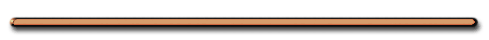HomeExplain This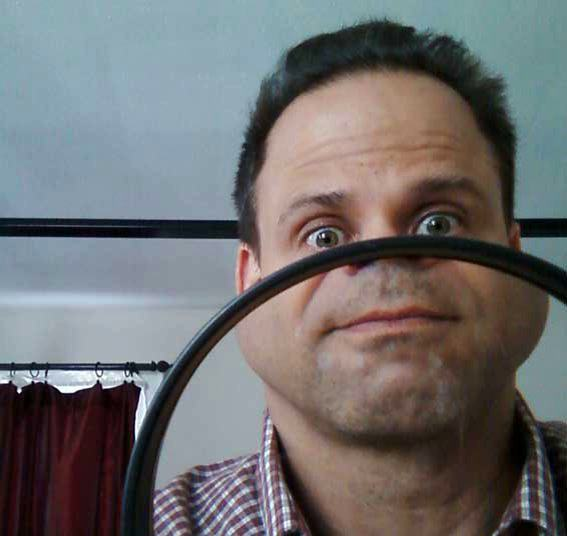The Physics of the Human Eye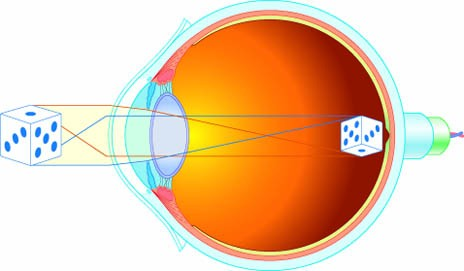Graphic from dorling kindersley books website

Video Instruction

showmethephysics.com

D. Index of Refraction (n) – a comparison of speed of light in a substance to its speed in a vacuum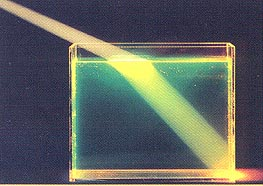If a substance has an index of refraction of 2.00 it means light is twice as fast in a vacuum than it is in that medium

 n = c/v

c = speed of light in a vacuum
(
3.00 x 108 m/s)

v = speed of light in a mediumnvacuum = 1.00

nair ≈ 1.00  , v ≈ 3 x 108 m/s

Which substance slows down light the most when light enters from air?

Diamond,

(highest (n) index of refraction)

least?

Water

(smallest (n) index of refraction)Any device with a lens refracts light

Ex 1) Speed of light in alcohol?

n = 1.36 (Reference Table)

n = 1.36 = c/v

1.36 = (3.00 x 108 m/s)/V

3.00 x 108 m/s = 1.36V

V = 2.21 x 108 m/s

Magnifying glasses enlarge objects with refraction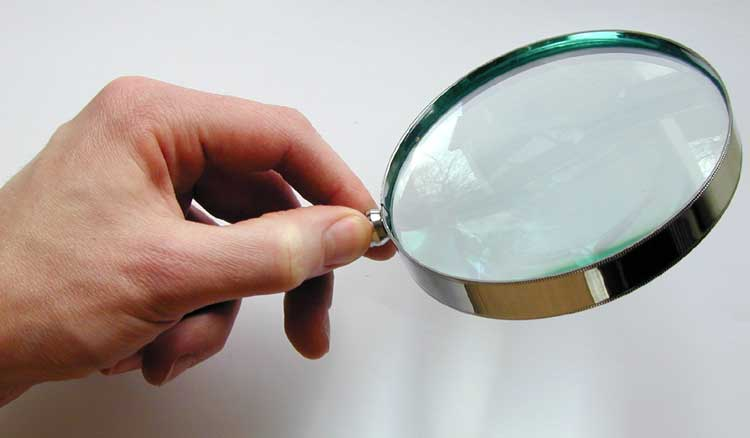n & v relationship?

n = c/v

Inverse

 The more light is  slowed down or sped up, the more it changes direction

Refraction in Glass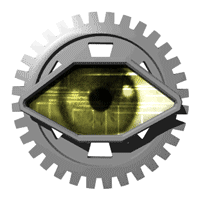The human eye refracts light

1. Prediction θref from θin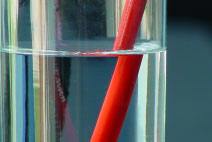a. Snell's Law

 n1sinθ1 = n2sinθ2

1 – incident medium

2 – refractive medium

Air/Lucite

n1sinθ1 = n2sinθ2

1.00 sin53 = (1.50) sinθ2

.798 = 1.50sinθ2

.798/1.50 = sinθ2

.532 = sinθ2

(inverse sine .532)

θ2 = 320

Ex) Light refracts as it moves from water to flint glass. Which angle is bigger, qin or qref?

Faster medium has bigger angle

Water 1.33?? or Flint glass 1.66??

Angle of Incidence bigger, Water faster

 Ranking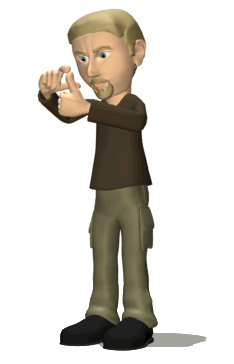Task Rank the media below from fastest to slowest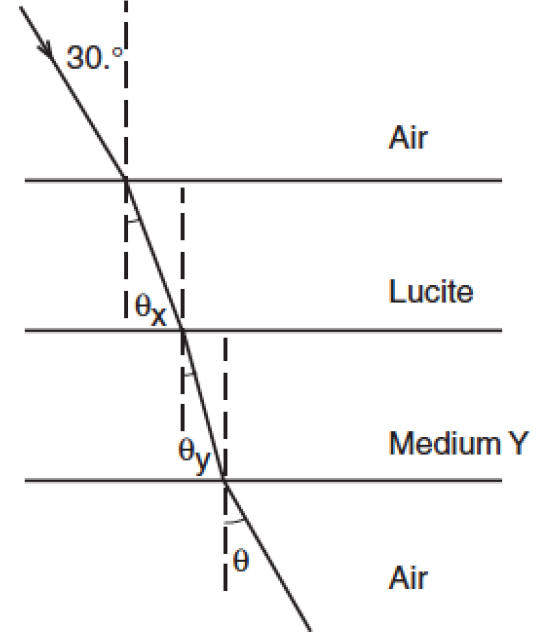Fooling Drinkers With RefractionRefraction RelationshipsEnrichment

NOVA (PBS) explains how refraction makes diamonds sparkle